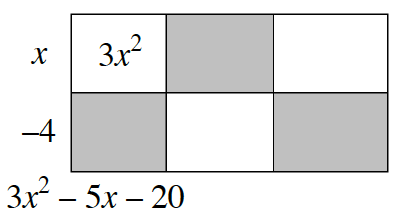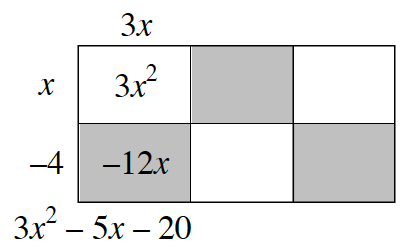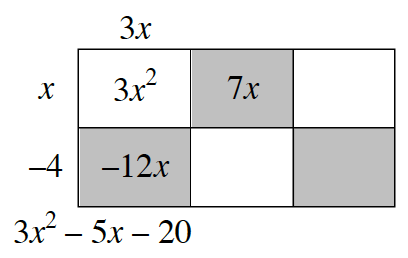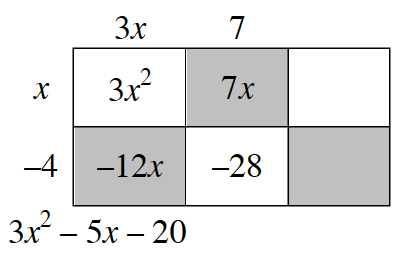### Home > PC3 > Chapter 4 > Lesson 4.2.2 > Problem4-82

4-82.

Use polynomial division to rewrite the equation $r(x)=\frac{3x^2-5x-20}{x-4}$ in a more-useful form. State the equations of the asymptotes. Then sketch a prediction of what you think the graph looks like. Use a graphing calculator to check you prediction.

Generic Rectangle, 2 rows, 3 columns, with expression, 3 x squared, minus 5 x, minus 20, below the rectangle. Left edge labeled, x minus 4, interior top left, labeled 3 x squared.

Labels added: top edge left, 3 x, interior bottom left, negative 12 x.

Label added: Interior top middle, 7 x.

Labels added: top edge middle, 7, interior bottom middle, negative 28.Continue the division problem. Part of the rewritten equation is a vertical asymptote, part is a slant asymptote.